Most Affordable JEE | NEET | 8,9,10 Preparation by Kota's Top IITian Doctor Faculties

# NCERT Solutions for Class 12 Physics Chapter 1 Electric Charge and Field PDF - eSaral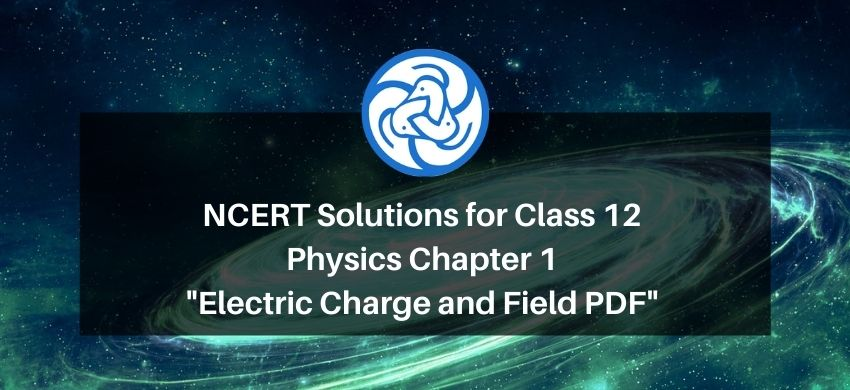`

# NCERT Solutions for Class 12 Physics Chapter 1 Electric Charge and Field PDF

Hey, are you a class 12 student and looking for ways to download NCERT Solutions for Class 12 Physics Chapter 1 Electric Charge and Field PDF? If yes. Then read this post till the end.

In this article, we have listed NCERT Solutions for Class 12 Physics Chapter 1 Electric Charge and Field in PDF that are prepared by Kota’s top IITian Faculties by keeping Simplicity in mind.

If you want to learn and understand class 12 Physics Chapter 1 "Electric Charge and Field" in an easy way then you can use these solutions PDF.

NCERT Solutions helps students to Practice important concepts of subjects easily. Class 12 Physics solutions provide detailed explanations of all the NCERT questions that students can use to clear their doubts instantly.

If you want to score high in your class 12 Physics Exam then it is very important for you to have a good knowledge of all the important topics, so to learn and practice those topics you can use eSaral NCERT Solutions.

In this article, we have listed NCERT Solutions for Class 12 Physics Chapter 1 Electric Charge and Field PDF that you can download to start your preparations anytime.

So, without wasting more time Let’s start.

### Download NCERT Solutions for Class 12 Physics Chapter 1 Electric Charge and Field PDF

Question 1. What is the force between two small charged sphere having charges of $2 \times$ $10^{-7} \mathrm{C}$ and $3 \times 10^{-7} \mathrm{C}$ placed $30 \mathrm{~cm}$ apart in air?

Solution. Given:

Charge on the first sphere, $\mathrm{Q}_{1}=2 \times 10^{-7} \mathrm{C}$

Charge on the first sphere, $\mathrm{Q}_{2}=3 \times 10^{-7} \mathrm{C}$

Distance between the spheres is, $r=30 \mathrm{~cm}, \mathrm{r}=0.3 \mathrm{~m}$

The electrostatic force between two charges is given by the formula, $\mathrm{F}=\frac{\mathrm{kQ}_{1} \mathrm{Q}_{2}}{\mathrm{r}^{2}}$

Where $\mathrm{k}=\frac{1}{4 \pi \varepsilon}=9 \times 10^{9}$

$\mathrm{F}=\frac{9 \times 10^{9} \times 2 \times 10^{-7} \times 3 \times 10^{-7}}{(0.3)^{2}}$

$=6 \times 10^{-3} \mathrm{~N}$

Hence, the force between the spheres is $6 \times 10^{-3} \mathrm{~N}$. Charges are of the same polarity so force between them will be repulsive in nature.

Question 2. The electrostatic force on a small sphere of charge $0.4 \mu \mathrm{C}$ due to another small sphere of charge $-0.8 \mu \mathrm{C}$ in the air is $0.2 \mathrm{~N}$.

(a) What is the distance between the two spheres?

(b) What is the force on the second sphere due to the first?

Solution. (a) Electrostatic force on first small sphere $=0.2 \mathrm{~N}$.

Charge on the first small sphere, $\mathrm{q}_{1}=0.4 \mu \mathrm{C}=0.4 \times 10^{-6} \mathrm{C}$

Charge on the second small sphere, $q_{2}=-0.8 \mu \mathrm{C}=0.8 \times 10^{-6} \mathrm{C}$

The electrostatic force between two small charges $=\frac{\mathrm{kQ}_{1} \mathrm{Q}_{2}}{\mathrm{r}^{2}}$

Where $\mathrm{k}=\frac{1}{4 \pi \varepsilon}=9 \times 10^{9}$

$r^{2}=\frac{k Q_{1} Q_{2}}{F}$

$=\frac{0.4 \times 10^{-6} \times 0.8 \times 10^{-6} \times 9 \times 10^{9}}{0.2}$

$=144 \times 10^{-4}$

$=0.12 \mathrm{~m}$

The distance between the two spheres is $0.12 \mathrm{~m}$.

(b) Both the sphere attracts each other with the same amount of force. Hence, the force on the second sphere will be $0.12 \mathrm{~m}$.

Question 3. Check that the ratio $\mathrm{ke}^{2} / \mathrm{Gm}_{\mathrm{e}} \mathrm{m}_{\mathrm{p}}$ is dimensionless. Look up a Table of Physical Constants and determine the value of this ratio. What does the ratio signify?

Solution. The given ratio is $=\frac{\mathrm{ke}^{2}}{\mathrm{Gm}_{\mathrm{e}} \mathrm{m}_{\mathrm{p}}}$

$\mathrm{m}_{\mathrm{e}}=$ mass of the electron

$\mathrm{m}_{\mathrm{p}}=$ mass of a proton

Unit of both the masses is $\mathrm{kg}$.

Where $\mathrm{G}=$ gravitational constant

Its unit is $\mathrm{N} \mathrm{m}^{2} \mathrm{~kg}^{-2}$

$\mathrm{e}=$ electric charge (Unit C)

where $\mathrm{k}=$ constant $\frac{1}{4 \pi \varepsilon}=9 \times 10^{9}$

$\varepsilon=$ permittivity of free space (Unit $\left.\mathrm{N} \mathrm{m}^{2} \mathrm{C}^{-2}\right)$

Unit of given ratio $=\frac{\mathrm{ke}^{2}}{\mathrm{Gm}_{\mathrm{e}} \mathrm{m}_{\mathrm{p}}}$

$=\frac{\left[\mathrm{N} \mathrm{m}^{2} \mathrm{C}^{-2}\right]\left[\mathrm{C}^{-2}\right]}{\left[\mathrm{N} \mathrm{m}^{2} \mathrm{Kg}^{-2}\right][\mathrm{Kg}][\mathrm{Kg}]}=\mathrm{M}^{\circ} \mathrm{L}^{\circ} \mathrm{T}^{\circ}$

Given ratio dimensionless

$\mathrm{e}=1.6 \times 10^{-19} \mathrm{C}$

$\mathrm{G}=6.67 \times 10^{-11} \mathrm{~N} \mathrm{~m}^{2} \mathrm{Kg}^{-2}$

$\mathrm{m}_{\mathrm{e}}=9.1 \times 10^{-31} \mathrm{~kg}$

$\mathrm{m}_{\mathrm{p}}=1.66 \times 10^{-27} \mathrm{~kg}$

Hence, the numerical value of the given ratio is

$\frac{\mathrm{ke}^{2}}{\mathrm{Gm}_{\mathrm{e}} \mathrm{m}_{\mathrm{p}}}=\frac{9 \times 10^{9} \times\left(1.6 \times 10^{-19}\right)^{2}}{6.67 \times 10^{-11} \times 9.1 \times 10^{-31} \times 1.66 \times 10^{-27}}$

$=2.3 \times 10^{39}$

Given is the ratios of electrostatic force to the gravitational force between a proton and an electron.

Question 4. (a) Explain the meaning of the statement 'electric charge of a body is quantized'.

(b) Why can one ignore the quantization of electric charge when dealing.

Solution. (a) The electric charge of a body is always quantized. A body possesses charge in an integral multiple of electronic charge. Only an integral number $(1,2,3 . . \mathrm{n})$ of electrons could be transferred from a body to another body, a fraction of charge could not be transferred. A body possesses charge in an integral multiple of electronic charge.

(b) On the macroscopic level, the number of charges used is too much then the magnitude of electronic charge. So, we ignore quantization of charge in on macroscopic level. We consider continuous charge on the macroscopic level.

Question 5. When a glass rod is rubbed with a silk cloth, charges appear on both. A similar phenomenon is observed with many other pairs of bodies. Explain how this observation is consistent with the law of conservation of charge.

Solution. When two bodies are rubbed with each other, charge of equal magnitude but opposite nature created on both the bodies. This is called charging by friction.

The net charge on two rubbed bodies will be zero. When a piece of glass rod is rubbed with a silk cloth, the charge appears on both, and the same phenomenon is observed in with many other pairs of bodies. This phenomenon is consistent with the law of conservation of charge.

Question 6. In a completely Four-point charges $q_{A}=2 \mu C, q_{B}=-5 \mu C, q_{c}=2 \mu C$, and $\mathrm{q}_{\mathrm{D}}=-5 \mu \mathrm{C}$ are located at the corners of a square $\mathrm{ABCD}$ of side $10 \mathrm{~cm}$. What is the force on a charge of $1 \mu C$ placed at the Centre of the square?

Solution. $\mathrm{AB}=\mathrm{BC}=\mathrm{CD}=\mathrm{AD}=10 \mathrm{~cm}$

$\mathrm{AC}=\mathrm{BD}=10 \sqrt{2} \mathrm{~cm}$

$\mathrm{AC}=\mathrm{OC}=\mathrm{DO}=\mathrm{OB}=5 \sqrt{2} \mathrm{~cm}$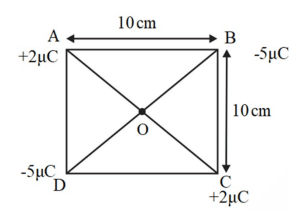The force applied by charge at corner $\mathrm{A}$ at centre $\mathrm{O}$ is equal in magnitude to the force applied by charge at $\mathrm{C}$ at center $\mathrm{O}$ but opposite in direction, so these two forces will cancel each other. The force applied by charge at corner $\mathrm{B}$ at center $\mathrm{O}$ is equal in magnitude to the force applied by charge at $\mathrm{D}$ at center $\mathrm{O}$ but opposite in direction, so these two forces will cancel each other. Hence, net force applied by the four charges is zero at center.

Question 7. (a) An electrostatic field line is a continuous curve. That is, a field line cannot have sudden breaks. Why not?

(b) Explain why two field lines never cross each other at any point?

Solution. A charge experiences a continuous force in the electric field so electric field line will be a continuous curve. The charge moves continuously, it does not jump. So, the electric field line cannot have sudden breaks.

Two field lines cannot cross each other because electric field intensity could not be in two directions at one point. Hence, two field lines cannot cross each other.

Question 8. Two-point charges $q_{A}=3 \mu C$ and $q_{B}=-3 \mu C$ are located $20 \mathrm{~cm}$ apart in vacuum.

(a) What is the electric field at the midpoint $\mathrm{O}$ of the line $\mathrm{AB}$ joining the two charges?

(b) If a negative test charge of magnitude $1.5 \times 10^{-9}$ Cis placed at this point, what is the force experienced by the test charge?

Solution. (a) As shown in the given figure. $\mathrm{O}$ is the mid $-$ point of line $\mathrm{AB}$.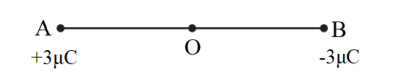(b)

Distance between the two charges, $A B=20 \mathrm{~cm}$

$\therefore \mathrm{AO}=\mathrm{OB}=10 \mathrm{~cm}$

The net electric field at point $0=\mathrm{E}$

Electric filed at point $\mathrm{O}$ caused by $+3 \mu \mathrm{C}$ charge is,

$E_{1}=\frac{3 \times 10^{6}}{4 \pi \varepsilon_{0}(A O)^{2}}$

$=\frac{3 \times 10^{-6}}{4 \pi \varepsilon_{0}\left(10 \times 10^{-2}\right)} \mathrm{N} / \mathrm{C}$

Along OB

Where, $\varepsilon_{0}=$ permittivity of free space

$\frac{1}{4 \pi \varepsilon_{0}}=9 \times 10^{9} \mathrm{~N} \mathrm{~m}^{2} \mathrm{C}^{2}$

The magnitude of the electric field at point $\mathrm{O}$ caused by $-3 \mu \mathrm{C}$ charge,

$\mathrm{E}_{2}=\left|\frac{-3 \times 10^{6}}{4 \pi \varepsilon_{0}(\mathrm{OB})^{2}}\right|=\left|\frac{3 \times 10^{-6}}{4 \pi \varepsilon_{0}\left(10 \times 10^{-2}\right)}\right| \mathrm{N} / \mathrm{C}$

Along OB

$\therefore \mathrm{E}=\mathrm{E}_{1}+\mathrm{E}_{2}$

$=2 \times\left[\left(9 \times 10^{9}\right) \times \frac{3 \times 10^{-6}}{\left(10 \times 10^{-2}\right)^{2}}\right]$

$=5.4 \times 10^{6} \mathrm{~N} \mathrm{C}^{-1}$ along $\mathrm{OB}$

[Since the values of $E_{1}$ and $E_{2}$ are same, the value is multiplied with 2 ]

Therefore, the electric field at point $\mathrm{O}$ is $5.4 \times 10^{6} \mathrm{~N} \mathrm{C}^{-1}$ along $\mathrm{OB}$.

(b) A test charge of amount $1.5 \times 10^{-9} \mathrm{C}$ is placed at mid- point $\mathrm{O}$.

$\mathrm{q}=1.5 \times 10^{-9} \mathrm{C}$

Force experienced by the test charge $=\mathrm{F}$

$\therefore \mathrm{F}=\mathrm{q} \mathrm{E}$

$=1.5 \times 10^{-9} \times 5.4 \times 10^{6}$

$\therefore \mathrm{F}=\mathrm{q} \mathrm{E}$

$=1.5 \times 10^{-9} \times 5.4 \times 10^{6}$

$=8.1 \times 10^{-3} \mathrm{~N}$

The force is directed along line $\mathrm{OA}$. This is because the negative test charge is repelled by the charge placed at point B but attracted towards point $\mathrm{A}$. Therefore, the force experienced by the test charge is $8.1 \times$ $10^{-3} \mathrm{~N}$ along $\mathrm{OA}$.

Question 9. A system has two charges $q_{\mathrm{A}}=2.5 \times 10^{-7} \mathrm{C}$ and $\mathrm{q}_{\mathrm{B}}=-2.5 \times 10^{-7} \mathrm{C}$ located at points A: $(0,0,-15 \mathrm{~cm})$ and B: $(0,0,+15 \mathrm{~cm})$, respectively. What is the total charge and electric dipole moment of the system?

Solution. The above situation can be represented as follows.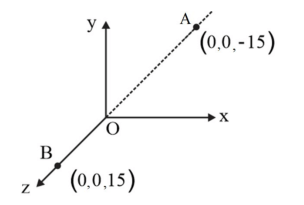At $\mathrm{A}$, amount of charge, $\mathrm{q}_{\mathrm{A}}=2.5 \times 10^{-7} \mathrm{C}$

At B, amount of charge, $q_{B}=-2.5 \times 10^{-7} \mathrm{C}$

Total charge of the system,

$\mathrm{q}=\mathrm{q}_{\mathrm{A}}+\mathrm{q}_{\mathrm{B}}$

$=2.5 \times 10^{7} \mathrm{C}-2.5 \times 10^{-7} \mathrm{C}$

$=0$

Distance between two charges which are present at points $\mathrm{A}$ and $\mathrm{B}$,

$\mathrm{d}=15+15=30 \mathrm{~cm}=0.3 \mathrm{~m}$

$\mathrm{d}=15+15=30 \mathrm{~cm}=0.3 \mathrm{~m}$

Electric dipole moment of the system is,

$p=q_{A} \times d=q_{B} \times d$

$=2.5 \times 10^{-7} \times 0.3$

$=7.5 \times 10^{-8} \mathrm{~cm}$ along positive $\mathrm{z}$ - axis

Therefore, the electric dipole moment of the system is $7.5 \times 10^{-8} \mathrm{~cm}$ along positive z-axis

Question 10. An electric dipole with dipole moment $4 \times 10^{-9} \mathrm{C} \mathrm{m}$ is aligned at $30^{\circ}$ with the direction of a uniform electric field of magnitude $5 \times 10^{4} \mathrm{NC}^{-1}$. Calculate the magnitude of the torque acting on the dipole.

Solution. Electric dipole moment, $\mathrm{p}=4 \times 10^{-9} \mathrm{~cm}$

Angle made by dipole $\mathrm{p}$ with a uniform electric field, $\theta=30^{\circ}$

Electric field, $\mathrm{E}=5 \times 10^{4} \mathrm{~N} \mathrm{C}^{-1}$

Torque acting on the dipole $\mathrm{p}$ is given by the relation,

$\mathrm{T}=\mathrm{p} \mathrm{E} \sin \theta$

$=4 \times 10^{-9} \times 5 \times 10^{4} \times \sin 30$

$=20 \times 10^{-5} \times \frac{1}{2}$

$=10^{-4} \mathrm{~N} \mathrm{~m}$

Therefore, the magnitude of the torque acting on the dipole $p$ is $10^{-4} \mathrm{~N} \mathrm{~m}$.

Question 11. A polythene piece rubbed with wool is found to have a negative charge of $3 \times 10^{-7} \mathrm{C}$

(a) Estimate the number of electrons transferred (from which to which?)

(b) Is there a transfer of mass from wool to polythene?

Solution. (a) When polythene is rubbed against wool, electrons get transferred from wool to polythene. Hence wool becomes positively charged and polythene becomes negatively charged.

Amount of net charge on the polythene piece, $q=-3 \times 10^{-7} \mathrm{C}$

Amount of charge on an electron, $\mathrm{e}=-1.6 \times 10^{-19} \mathrm{C}$

Total number of electrons got transferred from wool to polythene $=n$

n can be calculated using the formula,

$q=n e$

$n=\frac{-3 \times 10^{-7}}{-1.6 \times 10^{-19}}$

$\mathrm{n}=1.87 \times 10^{12}$

Therefore, the total number of electrons transferred from wool to polythene is $1.87 \times 10^{12}$

(b) Yes, There is a transfer of mass taking place. This is because an electron has mass, $\mathrm{m}_{\mathrm{e}}=9.1 \times 10^{-3} \mathrm{~kg}$

Total mass transferred to polythene from wool

$\mathrm{m}=\mathrm{m}_{\mathrm{e}} \times \mathrm{n}$

$=9.1 \times 10^{-31} \times 1.85 \times 10^{12}$

$=1.706 \times 10^{-18} \mathrm{~kg}$

Hence, a very small but negligible amount of mass is transferred from wool to polythene.

Question 12. (a) Two insulated charged copper spheres $\mathrm{A}$ and $\mathrm{B}$ have their centers separated by a distance of $50 \mathrm{~cm}$. What is the mutual force of electrostatic repulsion if the charge on each is $6.5 \times 10-7 \mathrm{C}$ ? The radii of $\mathrm{A}$ and $\mathrm{B}$ are negligible compared to the distance of separation.

(b) What is the force of repulsion if each sphere is charged double the above amount, and the distance between them is halved?

Solution. (a) Charge on sphere $\mathrm{A}, \mathrm{q}_{\mathrm{A}}=$ Charge on sphere $\mathrm{B}, \mathrm{q}_{\mathrm{B}}=6.5 \times 10^{-7} \mathrm{C}$

Distance between the spheres, $\mathrm{r}=50 \mathrm{~cm}=0.5 \mathrm{~m}$

Force of repulsion between the two spheres,

$F=\frac{q_{A} q_{B}}{4 \pi \varepsilon_{0} r^{2}}$

Where

$\varepsilon_{0}=$ free space permittivity

$\frac{1}{4 \pi \varepsilon_{0}}=9 \times 10^{9} \mathrm{~N} \mathrm{~m}^{2} \mathrm{C}^{-2}$

$\therefore \mathrm{F}=\frac{9 \times 10^{9} \times\left(6.5 \times 10^{-7}\right)^{2}}{(0.5)^{2}}$

$=1.52 \times 10^{-2} \mathrm{~N}$

Therefore, the net force between the two spheres is $1.52 \times 10^{-2} \mathrm{~N}$.

(b) If the charge is doubled, new charge on sphere $A, q_{A}=$ new charge on sphere $\mathrm{B}, \mathrm{q}_{\mathrm{B}}=2 \times 6.5 \times 10^{-7} \mathrm{C}=1.3 \times 10^{-6} \mathrm{C}$

The distance between the sphere is halved.

$\therefore \mathrm{r}=\frac{0.5}{2}=0.25 \mathrm{~m}$

Force of repulsion between the two spheres,

$\mathrm{F}=\frac{\mathrm{q}_{\mathrm{A}} \mathrm{q}_{\mathrm{B}}}{4 \pi \varepsilon_{0} \mathrm{r}^{2}}$

$\frac{=9 \times 10^{9} \times 1.3 \times 10^{-6} \times 1.3 \times 10^{-6}}{(0.25)^{2}}$

$=16 \times 1.52 \times 10^{-2}$

$=0.243 \mathrm{~N}$

Hence, the net force between the two spheres is $0.243 \mathrm{~N}$.

Question 13. Suppose the spheres A and B in Exercise $1.12$ have identical sizes. A third sphere of the same size but uncharged is brought in contact with the first, then brought in contact with the second, and finally removed from both. What is the new force of repulsion between $\mathrm{A}$ and $\mathrm{B}$ ?

Solution. Given:

Distance between the spheres, $\mathrm{A}$ and $\mathrm{B}, \mathrm{r}=0.5 \mathrm{~m}$

Initially, the charge on each sphere, $q=6.5 \times 10^{-7} \mathrm{C}$

When charged sphere $A$ with charge $q$ is touched with an uncharged sphere $C, \frac{q}{2}$ amount of charge from sphere A will transfer to sphere C. Hence, charge on each of the spheres, $\mathrm{A}$ and $\mathrm{C}$, is $\frac{\mathrm{q}}{2}$.

When sphere $\mathrm{C}$ with charge $\frac{\mathrm{q}}{2}$ is touched with sphere $\mathrm{B}$ with charge $\mathrm{q}$,

total charges of both spheres will divide into two equal halves given as,

$\frac{\frac{q}{2}+q}{2}=\frac{3 q}{4}$

Each sphere will share each half of charge. Hence, net charge on each of the spheres, $\mathrm{C}$ and $\mathrm{B}$, is $\frac{3 q}{4}$.

Force of repulsion between sphere A with charge $\frac{q}{2}$ and sphere B with charge

$\frac{3 q}{4}=\frac{\frac{9}{2} \times \frac{3 q}{4}}{4 \pi \varepsilon_{0} r^{2}}=\frac{3 q^{2}}{8 \times 4 \pi \varepsilon_{0} r^{2}}$

$=9 \times 10^{9} \times \frac{3 \times\left(6.5 \times 10^{-7}\right)^{2}}{8 \times(0.5)^{2}}$

$=5,703 \times 10^{-3} \mathrm{~N}$

Therefore, the force of attraction between the two spheres is $5.703 \times 10^{-3} \mathrm{~N}$.

Question 14. Figure $1.33$ shows tracks of three charged particles in a uniform electrostatic field. Give the signs of the three charges. Which particle has the highest charge to mass ratio?

Solution.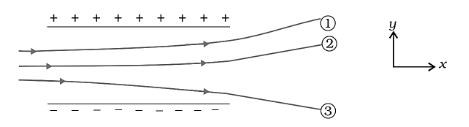Solution: Opposite charges attract each cither and same charges repel each other. Particles 1 and 2 both move towards the positively charged plate and repel away from the negatively charged plate. Hence, these two particles are negatively charged.

It can also be observed that particle 3 moves towards the negatively charged plate and repels away from the positively charged plate. Hence, particle 3 is positively charged.

The charge to mass ratio (emf) is directly proportional to the displacement or amount of deflection for a given velocity. Since the deflection of particle 3 is the maximum, it has the highest charge to mass ratio.

Question 15. Consider a uniform electric field $\mathrm{E}=3 \times 10^{3}$ î $\mathrm{N} / \mathrm{C}$.

(a) What is the flux of this field through a square of $10 \mathrm{~cm}$ on a side whose plane is parallel to the yz plane?

(b) What is the flux through the same square if the normal to its plane makes a $60^{\circ}$ angle with the $x$-axis?

Solution: (a) Given:

Electric field intensity, $\overrightarrow{\mathrm{E}}=3 \times 10^{3} \hat{\mathrm{N}} / \mathrm{C}$

Magnitude of electric field intensity, $|\overrightarrow{\mathrm{E}}|=3 \times 10^{3} \mathrm{~N} / \mathrm{C}$

Side of the square, $\mathrm{s}=10 \mathrm{~cm}=0.1 \mathrm{~m}$

Area of the square, $\mathrm{A}=\mathrm{s}^{2}=0.01 \mathrm{~m}^{2}$

The plane of the square is parallel to the $\mathrm{y}-\mathrm{z}$ plane. Hence, angle between the unit vector normal to the plane and electric field, $\theta=0^{\circ}$

Flux $(\phi)$ through the plane is given by the relation,

$\phi=|\overrightarrow{\mathrm{E}}| \mathrm{A} \cos \theta$

$=3 \times 10^{3} \times 0.01 \times \cos 0^{\circ}$

$=30 \mathrm{~N} \mathrm{~m}^{2} / \mathrm{C}$

(b) Plane makes an angle of $60^{\circ}$ with $\mathrm{x}$-axis. Hence, $\theta=60^{\circ}$

Flux, $\phi=|\overrightarrow{\mathrm{E}}| \mathrm{A} \cos \theta$

$=3 \times 10^{3} \times 0.01 \times \cos 60^{\circ}$

$=30 \times \frac{1}{2}=15 \mathrm{~N} \mathrm{~m}^{2} / \mathrm{C}$

Question 16. What is the net flux of the uniform electric field of Exercise $1.15$ through a cube of side $20 \mathrm{~cm}$ oriented so that its faces are parallel to the coordinate planes?

Solution. It is given that all the faces of a cube are parallel to the coordinate axes. Therefore, the number of field lines entering the cube is equal to the number of field lines coming out of the cube. As a result, net flux through the cube of $20 \mathrm{~cm}$ is zero.

Question 17. Careful measurement of the electric field at the surface of a black box indicates that the net outward flux through the surface of the box is $8.0 \times 10^{3} \mathrm{Nm}^{2} / \mathrm{C}$.

(a) What is the net charge inside the box?

(b) If the net outward flux through the surface of the box were zero, could you conclude that there were no charges inside the box? Why or Why not?

Solution. (a) Given:

Net outward flux through the surface of the box is $\phi=8.0 \times 10^{3} \mathrm{~N} \mathrm{~m}^{2} / \mathrm{C}$

For a body containing net charge $\mathrm{q}$, flux is given by the formula,

$\phi=\frac{q}{\varepsilon_{0}}$

$\varepsilon_{0}=$ permittivity of free space

$=8.854 \times 10^{-12} \mathrm{~N}^{-1} \mathrm{C}^{2} \mathrm{~m}^{-2}$

$\mathrm{q}=\varepsilon_{0} \phi$

$=8.854 \times 10^{-12} \times 8.0 \times 10^{3}$

$=7.08 \times 10^{-8}$

$=0.07 \mu \mathrm{C}$

Therefore, the net charge inside the box is $=0.07 \mu \mathrm{C}$

(b) No

Net flux coming out through a body depends on the presence of net charge contained in the body. If net flux is zero, it can be said that net charge inside the body is zero. The body may have equal amount of positive and negative charges.

Question 18. A point charge $+10 \mu \mathrm{C}$ is a distance $5 \mathrm{~cm}$ directly above the center of a square of side $10 \mathrm{~cm}$, as shown in Fig. $1.34$. What is the magnitude of the electric flux through the square? (Hint: Think of the square as one face of a cube with edge $10 \mathrm{~cm} .$ )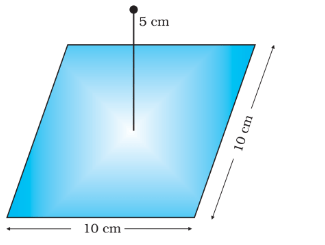Solution. A square could be considered as one face of a cube of side $10 \mathrm{~cm}$ with a center where charge $q$ is placed. According to Gauss's theorem, net electric flux is through all six faces is

$\phi_{\text {total }}=\frac{q}{\varepsilon_{0}}$

Hence, electric flux through one of the face of cube i.e., through the square face,

$=\frac{1}{6} \frac{q}{\varepsilon_{0}}$

Where

$\varepsilon_{0}=$ permittivity of free space

$=8.854 \times 10^{-12} \mathrm{~N}^{-1} \mathrm{C}^{2} \mathrm{~m}^{-2}$

$\mathrm{q}=10 \mu \mathrm{C}=10 \times 10^{-6} \mathrm{C}$

$\therefore \phi=\frac{1}{6} \times \frac{10 \times 10^{-6}}{8.854 \times 10^{-12}}$

$=1.88 \times 10^{5} \mathrm{~N} \mathrm{~m}^{2} \mathrm{C}^{-1}$

Therefore, electric flux through the square is $1.88 \times 10^{5} \mathrm{~N} \mathrm{~m}^{2} \mathrm{C}^{-1}$.

Question 19. A point charge of $2.0 \mu \mathrm{C}$ is at the center of a cubic Gaussian surface $9.0 \mathrm{~cm}$ on edge. What is the net electric flux through the surface?

Solution. Net electric flux $\left(\phi_{\text {Net }}\right)$ through the cubic surface is given by,

$\phi_{\text {Net }}=\frac{q}{\varepsilon_{0}}$

$\varepsilon_{0}=$ permittivity of free space

$=8.854 \times 10^{-12} \mathrm{~N}^{-1} \mathrm{C}^{2} \mathrm{~m}^{-2}$

$\mathrm{q}=$ Net charge contained inside the cube $=2.0 \mu \mathrm{C}=2 \times 10^{-6} \mathrm{C}$

$\phi_{\mathrm{Net}}=\frac{2 \times 10^{-6}}{8.854 \times 10^{-12}}$

$=2.26 \times 10^{5} \mathrm{~N} \mathrm{~m}^{2} \mathrm{C}^{-1}$

The net electric flux through the surface of cube is $2.26 \times 10^{5} \mathrm{~N} \mathrm{~m}^{2} \mathrm{C}^{-1}$.

Question 20. A point charge causes an electric flux of $-1.0 \times 103 \mathrm{Nm} 2 / \mathrm{C}$ to pass through a spherical Gaussian surface of $10.0 \mathrm{~cm}$ radius centered on the charge.

(a) If the radius of the Gaussian surface were doubled, how much flux would pass through the surface?

(b) What is the value of the point charge?

Solution. (a) Electric flux, $\phi=-1.0 \times 10^{3} \mathrm{~N} \mathrm{~m}^{2} / \mathrm{C}$

$\mathrm{r}-10.0 \mathrm{~cm}$

Electric Flux coming out through a surface depends on the net charge contained inside a body. It does not depend on the size of the body. If the radius of the Gaussian surface is doubled, then the net Flux passing through the surface remains the Same i. e., $-10^{3} \mathrm{~N} \mathrm{~m}^{2} / \mathrm{C}$

(b) Electric Flux is given by the formula,

$\phi=\frac{\mathrm{q}}{\varepsilon_{0}}$

Where,

$\mathrm{q}=$ Net charge contained by the spherical surface

$\varepsilon_{0}=$ Permittivity of free space $=8.854 \times 10^{-12} \mathrm{~N}^{-1} \mathrm{C}^{2} \mathrm{~m}^{-1}$

$\therefore \mathrm{q}=\phi \varepsilon_{0}$

$=-1.0 \times 10^{3} \times 8.854 \times 10^{-12}$

$=-8.854 \times 10^{-9} \mathrm{C}$

$=-8.854 \mathrm{nC}$

Therefore, the value of the point charge is $-8.854 \mathrm{nC}$.

Question 21. A conducting sphere of radius $10 \mathrm{~cm}$ has an unknown charge. If the electric field $20 \mathrm{~cm}$ from the center of the sphere is $1.5 \times 10^{3} \mathrm{~N} / \mathrm{C}$ and points radially inward, what is the net charge on the sphere?

Solution. Electric field intensity (E) at a distance (d) from the center of a sphere containing net charge $q$ is,

$E=\frac{q}{4 \pi \varepsilon_{0} d^{2}}$

Where.

$\mathrm{q}=$ Net charge $=1.5 \times 10^{3} \mathrm{~N} / \mathrm{C}$

$\mathrm{d}=$ Distance from the center of sphere $=20 \mathrm{~cm}=0.2 \mathrm{~m}$

$\varepsilon_{0}=$ permittivity of free space

And $\frac{1}{4 \pi \varepsilon_{0}}=9 \times 10^{9} \mathrm{~N} \mathrm{~m}^{2} \mathrm{C}^{-2}$

$q=E\left(4 \pi \varepsilon_{0}\right) d^{2}$

$=\frac{1.5 \times 10^{3} \times(0.2)^{2}}{9 \times 10^{9}}$

$=6.76 \times 10^{9} \mathrm{C}$

$=6.67 \mathrm{nC}$

Therefore, the net charge on the sphere is $6.67 \mathrm{nC}$

Question 22. A uniformly charged conducting sphere of $2.4 \mathrm{~m}$ diameter has a surface charge density of $80.0 \mu \mathrm{C} / \mathrm{m}^{2}$.

(a) Find the charge on the sphere.

(b) What is the total electric flux leaving the surface of the sphere?

Solution. (a) Diameter of the sphere, $\mathrm{d}=2.4 \mathrm{~m}$

Radius of the sphere, $\mathrm{r}=1.2 \mathrm{~m}$

Surface charge density, $\sigma=80.0 \mu \mathrm{C} / \mathrm{m}^{2}=80 \times 10^{-6} \mathrm{C} / \mathrm{m}^{2}$

Total charge on the surface of the sphere,

$\mathrm{Q}=$ Charge density $\times$ Surface area

$=\sigma \times 4 \pi r^{2}$

$=80 \times 10^{-6} \times 4 \times 3.14 \times(1.2)^{2}$

$=1.447 \times 10^{-3} \mathrm{C}$

Therefore, the charge on the sphere is $1.447 \times 10^{-3} \mathrm{C}$.

(b) Total electric flux $\left(\phi_{\text {total }}\right)$ passing out the surface of a sphere containing net charge $\mathrm{Q}$ is given by the relation,

$\phi_{\text {total }}=\frac{Q}{\varepsilon_{0}}$

Where,

$\varepsilon_{0}=$ permittivity of free space

$=8.854 \times 10^{-12} \mathrm{~N}^{-1} \mathrm{C}^{2} \mathrm{~m}^{-2}$

$\mathrm{Q}=1.447 \times 10^{-3} \mathrm{C}$

$\phi_{\text {total }}=\frac{1.44 \times 10^{-3}}{8.854 \times 10^{-12}}$

$=1.63 \times 10^{8} \mathrm{~N} \mathrm{C}^{-1} \mathrm{~m}^{2}$

Therefore, the total electric flux passing the surface of the sphere is $1.63 \times 10^{9} \mathrm{~N} \mathrm{C}^{-1} \mathrm{~m}^{2}$

Question 23. An infinite line charge produces a field of $9 \times 104 \mathrm{~N} / \mathrm{C}$ at a distance of $2 \mathrm{~cm}$. Calculate the linear charge density.

Solution. Electric field by the infinite line charges have linear charge density $\lambda$ at a distance $\mathrm{d}$ is,

$\mathrm{E}=\frac{\lambda}{2 \pi \varepsilon_{0} \mathrm{~d}}$

$\Rightarrow \lambda=2 \pi \varepsilon_{0} \mathrm{Ed}$

Where,

$\mathrm{d}=2 \mathrm{~cm}=0.02 \mathrm{~m}$

$\mathrm{E}=9 \times 10^{4} \mathrm{~N} / \mathrm{C}$

$\varepsilon_{0}=$ permittivity of free space

$\frac{1}{4 \pi \varepsilon_{0}}=9 \times 10^{9} \mathrm{~N} \mathrm{~m}^{2} \mathrm{C}^{-2}$

$\therefore \lambda=\frac{0.02 \times 9 \times 10^{4}}{2 \times 9 \times 10^{9}}=10 \mu \mathrm{C} / \mathrm{m}$

Therefore, the linear charge density is $10 \mu \mathrm{C} / \mathrm{m}$.

Question 24. Two large, thin metal plates are parallel and close to each other. On their inner faces, the plates have surface charge densities of opposite signs and of magnitude $17.0 \times 10^{-22} \mathrm{C} / \mathrm{m}^{2}$. What is $\mathrm{E}$ :

(a) in the outer region of the first plate,

(b) in the outer region of the second plate, and

(c) between the plates?

Solution. The situation is represented by the following figure.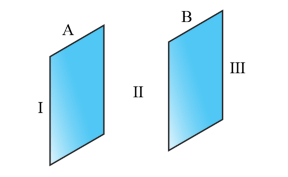$\mathrm{A}$ and $\mathrm{B}$ are two parallel plates close to ea. other. Outer region of plate $\mathrm{A}$ is labelled as I, outer region of plate $\mathrm{B}$ is labelled as III, and the region between the plates, $\mathrm{A}$ and 0, is labelled as II.

Charge density of plate $\mathrm{A}, \sigma=17.0 \times 10^{-22} \mathrm{C} / \mathrm{m}^{2}$

Charge density of plate $\mathrm{B}, \sigma=17.0 \times 10^{-22} \mathrm{C} / \mathrm{m}^{2}$

In the regions, I and III, electric field $\mathrm{E}$ is zero because charge is not enclosed by the respective plates.

Electric field $\mathrm{E}$ in region II is,

$\mathrm{E}=\frac{\sigma}{\varepsilon_{0}}$

Where, $\varepsilon_{0}=$ permittivity of free space $=8.854 \times 10^{-12} \mathrm{~N}^{-1} \mathrm{C}^{2} \mathrm{~m}^{-2}$

$\mathrm{E}=\frac{17.0 \times 10^{22}}{8.854 \times 10^{-12}}$

$=1.92 \times 10^{-19} \mathrm{~N} / \mathrm{C}$

Therefore, electric field between the plates is $1.92 \times 10^{-19} \mathrm{~N} / \mathrm{C}$

Question 25. An oil drop of 12 excess electrons is held stationary under a constant electric field of $2.55 \times 10^{4} \mathrm{~N} \mathrm{C}^{-1}$ (Millikan's oil drop experiment). The density of the oil is $1.26 \mathrm{~g} \mathrm{~cm}^{-3} .$

Estimate the radius of the drop. $\left(\mathrm{g}=9.81 \mathrm{~m} \mathrm{~s}^{-2} ; \mathrm{e}=1.60 \times 10^{-19} \mathrm{C}\right)$.

Solution: Given:

Excess electrons on an oil drop, $\mathrm{n}=12$

Electric field intensity, $\mathrm{E}=2.55 \times 10^{4} \mathrm{~N} \mathrm{C}^{-1}$

Density of oil, $\rho=1.26 \mathrm{gm} / \mathrm{cm}^{3}=1.26 \times 10^{3} \mathrm{gm} / \mathrm{kg}^{3}$

Acceleration due to gravity, $\mathrm{g}=9.81 \mathrm{~m} \mathrm{~s}^{-2}$

Charge on an electron, $\mathrm{e}=1.6 \times 10^{-19} \mathrm{C}$

Radius of the oil drop $=\mathrm{r}$

Force (F) on oil drop due to electric field $E$ is equal to the weight of the oil drop $(\mathrm{W})$

$\mathrm{F}=\mathrm{W}$

$\Rightarrow \mathrm{qE}=\mathrm{mg}$

$\Rightarrow n e E=\frac{4}{3} \pi r^{3} \times \rho \times g$

$\mathrm{q}=$ Net charge on the oil drop $=\mathrm{ne}$

$\mathrm{m}=$ Mass of the oil drop

$=$ Volume of the oil drop $\times$ Density of oil $=\frac{4}{3} \pi r^{3} \times \rho$

$\therefore \mathrm{r}==\sqrt{\frac{3 \mathrm{Ene}}{4 \pi \rho \mathrm{g}}}$

$=\sqrt{\frac{3 \times 2.55 \times 10^{4} \times 12 \times 1.6 \times 10^{-19}}{4 \times 3.14 \times 1.26 \times 10^{3} \times 9.81}}$

$=\sqrt{946.09 \times 10^{-21}}$

$=\sqrt{946.09 \times 10^{-21}}$

$=9.82 \times 10^{-7} \mathrm{~m}$

Therefore, the radius of the oil drop is $9.82 \times 10^{-4} \mathrm{~mm}$.

Question 26. Which among the curves shown in Fig. $1.35$ cannot possibly represent electrostatic field lines?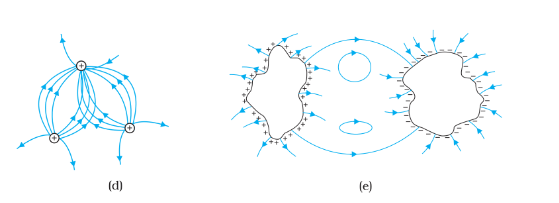Solution: (a) In figure (a), field lines shown do not represent electrostatic field lines because field lines could not be at any random angle to surface, it must be normal to the surface of the conductor.

(b) In figure (b), field lines shown do not represent electrostatic field lines because the field lines cannot emerge from a negative charge and cannot terminate at a positive charge.

(c) In figure (c), field lines shown represent electrostatic field lines because the field lines emerge from the positive charges and repel each other.

(d) In figure (d), field lines shown do not represent electrostatic field lines because the field lines should not intersect each other.

(e) In figure (e), field lines shown do not represent electrostatic field lines because closed loops are not formed in the area between the field lines.

Question 27. In a certain region of space, electric field is along the z-direction throughout. The magnitude of the electric field is, however, not constant but increases uniformly along the positive z-direction, at the rate of $105 \mathrm{~N} \mathrm{C}^{-1}$ per meter. What are the force and torque experienced by a system having a total dipole moment equal to $10^{-7} \mathrm{Cm}$ in the negative z-direction?

Solution. Dipole moment of the system, $\mathrm{p}=\mathrm{q} \times \mathrm{dl}=-10^{-7} \mathrm{C} \mathrm{m}$

\text { Rate of increase of electric field per unit length, }

$\frac{\mathrm{dE}}{\mathrm{dl}}=10^{-5} \mathrm{~N} \mathrm{C}^{-1}$

Force (F) experienced by the system is,

$\mathrm{F}=\mathrm{qE}$

$\mathrm{F}=\mathrm{q} \frac{\mathrm{dE}}{\mathrm{dl}} \times \mathrm{dl}$

$=p \times \frac{d E}{d l}$

$=-10^{-7} \times 10^{-5}$

$=-10^{-2} \mathrm{~N}$

The force is $10^{-2} \mathrm{~N}$ in the negative z-direction i.e., opposite to the direction of electric field. Hence, the angle between electric field and dipole moment is $180^{\circ}$. Torque (T) given by the relation,

$\mathrm{T}=\mathrm{pE} \sin 180^{\circ}$

$=0$

Therefore, the torque experienced by the system is zero.

Question 28. (a) A conductor A with a cavity as shown in Fig. $1.36(\mathrm{a})$ is given a charge $\mathrm{Q}$. Show that the entire charge must appear on the outer surface of the conductor.

(b) Another conductor B with charge $q$ is inserted into the cavity keeping B insulated from $\mathrm{A}$. Show that the total charge on the outside surface of $\mathrm{A}$ is $\mathrm{Q}+\mathrm{q}[\mathrm{Fig} .1 .36(\mathrm{~b})]$

(c) A sensitive instrument is to be shielded from the strong electrostatic fields in its environment. Suggest a possible way.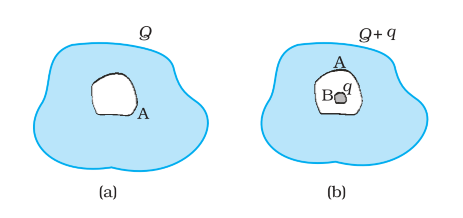Solution: (a) Let us consider a Gaussian surface that is lying within a conductor and having the cavity. The electric field intensity $\mathrm{E}$ inside the charged conductor is zero.

Let $\mathrm{q}$ is the charge inside the conductor and $\varepsilon_{0}$ is the permittivity of free space.

Flux $\phi=\overrightarrow{\mathrm{E}} \cdot \overrightarrow{\mathrm{ds}}=\frac{\mathrm{q}}{\varepsilon_{0}}$

Here, $\mathrm{E}=0$

$\frac{\mathrm{q}}{\varepsilon_{0}}=0$

$\therefore \varepsilon_{0} \neq 0$

$\therefore \mathrm{q}=0$

Therefore, charge inside the conductor is zero so entire charge $Q$ appears on the outer surface of the conductor.

(b) The outer surface of conductor A has a charge of amount $Q$. Another conductor B having charge $+\mathrm{q}$ is kept inside conductor $\mathrm{A}$ and it is insulated from $\mathrm{A}$. Hence, a charge of amount $-\mathrm{q}$ will be induced in the inner surface of conductor $\mathrm{A}$ and $+\mathrm{q}$ is induced on the outer surface of conductor $A$. Therefore, total charge on the outer surface of conductor $A$ is $\mathrm{Q}+\mathrm{q}$

(c) A sensitive instrument can be shielded from the strong electrostatic field in its environment by enclosing it fully inside a metallic surface. A closed metallic body acts as an electrostatic shield.

Question 29. A hollow charged conductor has a tiny hole cut into its surface. Show that the electric field in the hole is $\left(\frac{\sigma}{2 \varepsilon_{0}}\right) \hat{n}$, where $\hat{n}$ is the unit vector in the outward normal direction, and $\sigma$ is the surface charge density near the hole.

Solution. Let us consider a conductor with a Cavity. Electric inside the cavity is Zero.

Let $\mathrm{E}$ is the electric field just outside the conductor, $\mathrm{q}$ is the electric charge, $\sigma$ is the charge density, and $\varepsilon_{0}$ is the permittivity of free space.

Charge $|\mathrm{q}|=\vec{\sigma} \times \overrightarrow{\mathrm{ds}}$

According to Gauss's law,

Flux, $\phi=\overrightarrow{\mathrm{Eds}}=\frac{|\mathrm{q}|}{\varepsilon_{0}}$

$\Rightarrow \mathrm{Eds}=\frac{\vec{\sigma} \times \overrightarrow{\mathrm{ds}}}{\varepsilon_{0}}$

$\therefore \mathrm{E}=\frac{\sigma}{\varepsilon_{0}} \hat{\mathrm{n}}$

Therefore, the electric field must outside the conductor is $\frac{\sigma}{\varepsilon_{0}} \hat{n} .$ This field is a superposition of field due to the cavity (E') and the field due to the rest of the charged conductor (E'). These fields are equal and opposite inside the conductor, and equal in magnitude and direction outside the conductor.

$\therefore \mathrm{E}^{\prime}+\mathrm{E}^{\prime}=\mathrm{E}$

$\mathrm{E}^{\prime}=\frac{\mathrm{E}}{2}$

$=\frac{\sigma}{2 \varepsilon_{0}} \mathrm{n}$

Therefore, the field due to the rest of the conductor is $\frac{\sigma}{\varepsilon_{0}} n$.

Question 30. Obtain the formula for the electric field due to a long thin wire of uniform linear charge density $\mathrm{E}$ without using Gauss's law. [Hint: Use Coulomb's law directly and evaluate the necessary integral.]

Solution. Take along thin wire XY (as shown in figure) of uniform linear charge density $\lambda$.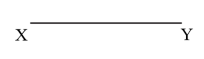Consider a point $\mathrm{A}$ at a perpendicular distance $\mathrm{l}$ from the mid-point $\mathrm{O}$, as shown in the figure.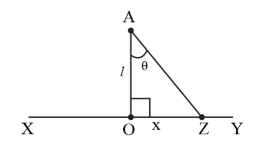Let $\mathrm{E}$ be the electric field at point $\mathrm{A}$ due to the wire, $\mathrm{XY}$.

Take a small length element $\mathrm{dx}$ on the wire with $\mathrm{OZ}=x$

Let $q$ be the charge on this element.

$\therefore \mathrm{q}=\lambda \mathrm{d} x$

Electrical field due to the element,

$\mathrm{DE}=\frac{1}{4 \pi \varepsilon_{0}} \frac{\lambda \mathrm{d} x}{(\mathrm{AZ})^{2}}$

However, $A Z=\sqrt{\left(1^{2}+x^{2}\right)}$

$\therefore \mathrm{dE}=\frac{\lambda \mathrm{dx}}{4 \pi \varepsilon_{0}\left(1^{2}+\mathrm{x}^{2}\right)}$

The electric field is resolved into two different rectangular components. $\mathrm{dEcos} \theta$ is the perpendicular component of electric field and $\mathrm{dEsin} \theta$ is the parallel component of electric field.

When the whole wire is considered, the small component $\mathrm{dEsin} \theta$ is cancelled.

Only the perpendicular component of electric field $\mathrm{dE} \cos \theta$ affects point $\mathrm{A}$.

Hence, effective electric field at point A due to the small element $\mathrm{dx}$ is $\mathrm{dE}_{1}$.

$\therefore \mathrm{dE}_{1}=\frac{\lambda \mathrm{dxcos} \theta}{4 \pi \varepsilon_{0}\left(x^{2}+1^{2}\right)}$ $\ldots \ldots . . .$ (1)

In $\Delta \mathrm{AZO}$,

$\tan \theta=\frac{x}{1}$

$\Rightarrow x=1 \times \tan \theta$ $\ldots \ldots(2)$

On differentiating equation (2), we obtain

$\frac{\mathrm{d} x}{\mathrm{~d} \theta}=\operatorname{lsin}^{2} \theta$

$\Rightarrow \mathrm{dx}=\operatorname{lsin}^{2} \theta \mathrm{d} \theta$ $\ldots . .$ (3)

From equation (2), $x^{2}+l^{2}=l^{2}+l^{2} \tan ^{2} \theta$

$\Rightarrow 1^{2}\left(1+\tan ^{2} \theta\right)=l^{2} \sec ^{2} \theta$

$\Rightarrow x^{2}+1^{2}=1^{2} \sin ^{2} \theta$ $\ldots . .(4)$

Putting equations (3) and (4) in equation (1), we obtain

$\therefore \mathrm{dE}_{1}=\frac{\lambda \operatorname{lsec}^{2} \mathrm{~d} \theta}{4 \pi \varepsilon_{0} 1^{2} \sec ^{2} \theta} \times \cos \theta$

$\therefore \mathrm{dE}_{1}=\frac{\lambda \operatorname{scos}^{2} \mathrm{~d} \theta}{4 \pi \varepsilon_{0} 1}$

$\therefore \mathrm{dE}_{1}=\frac{\lambda \operatorname{scos}^{2} \mathrm{~d} \theta}{4 \pi \varepsilon_{0} 1}$ $\ldots \ldots$ (5)

The wire is so long so $\theta$ tends from $-\frac{\pi}{2}$ to $+\frac{\pi}{2}$.

By integrating equation (5), the obtained the value of field $\mathrm{E}_{1}$ as,

$\int_{-\frac{\pi}{2}}^{\frac{\pi}{2}} \mathrm{~d} \mathrm{E}_{1}=\int_{-\frac{\pi}{2}}^{\frac{\pi}{2}} \frac{\lambda}{4 \pi \varepsilon_{0} 1} \cos \theta \mathrm{d} \theta$

$\mathrm{E}_{1}=\frac{\lambda}{4 \pi \varepsilon_{0} 1}[\sin \theta]_{-\frac{\pi}{2}}^{\frac{\pi}{2}}=\frac{\lambda}{4 \pi \varepsilon_{0} 1} \times 2$

$\Rightarrow \mathrm{E}_{1}=\frac{\lambda}{2 \pi \varepsilon_{0} 1}$

Therefore, the electric field due to long wire is $\frac{\lambda}{2 \pi \varepsilon_{0} 1}$

Question 31. It is now believed that protons and neutrons (which constitute nuclei of ordinary matter) are themselves built out of more elementary units called quarks. A proton and a neutron consist of three quarks each. Two types of quarks, the so called 'up' quark (denoted by u) of charge $+(2 / 3) \mathrm{e}$, and the 'down' quark (denoted by d) of charge $(-1 / 3)$ e, together with eleotrons build up ordinary matter. (Quarks-of other types have also been found which give rise to different unusual varieties of matter.) Suggest a possible quark composition of a proton and neutron.

Solution. A proton has three quarks. Let there be $\mathrm{n}$ up quarks in a proton, each having a charge of $+\frac{2}{3} e$

Charge due to $\mathrm{n}$ up quarks is $+\left(\frac{2}{3} \mathrm{e}\right) \mathrm{n}$

Number of down quarks has charge $-\frac{1}{3} \mathrm{e}$

Charge due to $(3-\mathrm{n})$ down quarks $=\left(-\frac{1}{3} \mathrm{e}\right)(3-\mathrm{n})$

Total charge on proton $=+\mathrm{e}$

$\therefore \mathrm{e}=\left(\frac{2}{3} \mathrm{e}\right) \mathrm{n}+\left(-\frac{1}{3} \mathrm{e}\right) 3-\mathrm{n}$

$\Rightarrow \mathrm{e}=\left(\frac{2 \mathrm{ne}}{3}\right)-\mathrm{e}+\frac{\mathrm{ne}}{3}$

$\Rightarrow 2 \mathrm{e}=\mathrm{ne}$

$\Rightarrow \mathrm{n}=2$

Number of up quarks in a proton, $\mathrm{n}=2$

Number of down quarks in a proton $=3-\mathrm{n}-3-2-\mathrm{I}$

Therefore, a proton can be represented as 'uud'.

A neutron also has three quarks. Let there be $\mathrm{n}$ up quarks in a neutron, each having

a charge of $+\frac{3}{2} \mathrm{e}$.

Charge on a neutron due to $\mathrm{n}$ up quarks $=\left(+\frac{3}{2} \mathrm{e}\right) \mathrm{n}$

Number down quarks is ( $3-\mathrm{n})$ each having a charge of $-\frac{1}{3} \mathrm{e}$

Charge on a neutron due to $(3-\mathrm{n})$ down quarks $=\left(-\frac{3}{2} \mathrm{e}\right)(3-\mathrm{n})$

Total charge on a neutron $=0$

$0=\left(\frac{2}{3} \mathrm{e}\right) \mathrm{n}+\left(-\frac{1}{3} \mathrm{e}\right)(3-\mathrm{n})$

$\Rightarrow 0=\frac{2}{3}$ en $-e+\frac{n e}{3}$

$\Rightarrow \mathrm{e}=\mathrm{ne}$

$\Rightarrow \mathrm{n}=1$

Number of up quarks in a neutron, $\mathrm{n}=1$

Number of down quarks in a neutron $=3-\mathrm{n}=2$

Therefore, a neutron can be represented as 'uud'.

Question 32. (a) Consider an arbitrary electrostatic field configuration. A small test charge is placed at a point (i.e., where $\mathrm{E}=0$ ) of the configuration. Show that the equilibrium of the test charge is necessarily unstable.

(b) Verify this result for the simple configuration of two charges of the same magnitude and sign placed a certain distance apart.

Solution. (a) Let the equilibrium of the test charge be stable. If test charge is in equilibrium and it is displaced from its position in any direction, then it experiences a force towards a point, where the electric field is zero. All the field lines near the point are directed inwards and towards the point. There is a net inward flux through a closed surface around the point. According to Gauss's law, the flux of electric field through a surface, which is not enclosing any charge, is zero. Hence, the equilibrium of the test charge can be stable.

(b) Two charges of same magnitude and same sign are placed at some distance. The mid-point of the joining line of the two charges is the point. When a test charged is displaced along the line joining charges, it experiences a restoring force. If it is displaced normal to the joining line of charges, then the net force takes it away from the point. Hence, the charge is not stable because stability of equilibrium requires restoring force in all directions.

Question 33. A particle of mass $\mathrm{m}$ and charge $(-\mathrm{q})$ enters the region between the two charged plates initially moving along $x$-axis with speed $v_{\mathrm{x}}$ (like particle 1 in Fig. 1.33). The length of plate is $\mathrm{L}$ and an uniform electric field $\mathrm{E}$ is maintained between the plates. Show that the vertical deflection of the particle at the far edge of the plate is $\mathrm{qEL}^{2} /\left(2 \mathrm{~m} v_{x}^{2}\right)$. Compare this motion with motion of a projectile in gravitational field discussed in Section $4.10$ of Class XI Textbook of Physics.

Solution. Charge on a particle of mass $\mathrm{m}=-\mathrm{q}$

Velocity of the particle $=\mathrm{v}_{x}$

Length of the plates $=\mathrm{L}$

Magnitude of the uniform electric field between the plates $=\mathrm{E}$

Mechanical force, $\mathrm{F}=$ Mass $(\mathrm{m}) \times$ Acceleration (a)

$\mathrm{a}=\frac{\mathrm{F}}{\mathrm{m}}$

However, electric force, $\mathrm{F}=\mathrm{qE}$

Therefore, acceleration of particle, $a=\frac{q E}{m} \ldots \ldots$ (1)

Total time taken by the particle to cross the electric field of length $\mathrm{L}$ is given by,

$\mathrm{t}=\frac{\text { Length of plate }}{\text { Velocity of the particle }}=\frac{\mathrm{L}}{\mathrm{v}_{\mathrm{x}}} \ldots \ldots$ (2)

In the vertical direction, initial velocity of particle, $\mathrm{u}=0$

According to the equation of motion, vertical deflection s of the particle is,

$\mathrm{s}=\mathrm{ut}+\frac{1}{2} \mathrm{at}^{2}$

$\mathrm{s}=0+\frac{1}{2}\left(\frac{\mathrm{qE}}{\mathrm{m}}\right)\left(\frac{\mathrm{L}}{\mathrm{V}_{\mathrm{x}}}\right)^{2}$

$\mathrm{S}=\frac{\mathrm{qEL}^{2}}{2 \mathrm{mV}_{\mathrm{x}}^{2}} \ldots \ldots$ (3)

Hence, vertical deflection of the particle at the far edge of the plate is $\mathrm{qEL}^{2} /$ $\left(2 \mathrm{mv}_{x}^{2}\right)$

This is like the motion of horizontal projectiles under gravity.

Question 34. Suppose that the particle in Exercise in $1.33$ is an electron projected with velocity $\mathrm{v}_{\mathrm{x}}=2.0 \times 10^{6} \mathrm{~m} \mathrm{~s}^{-1} .$ If $\mathrm{E}$ between the plates separated by $0.5 \mathrm{~cm}$ is $9.1 \times$ $10^{2} \mathrm{~N} / \mathrm{C}$, where will the electron strike the upper plate? $(|\mathrm{e}|=1.6 \times$ $\left.10^{-19} \mathrm{C}, \mathrm{m}_{\mathrm{e}}=9.1 \times 10^{-31} \mathrm{~kg} .\right)$

Solution. Velocity of the particle, $\mathrm{v}_{\mathrm{x}}=2.0 \times 10^{6} \mathrm{~m} / \mathrm{s}$

Separation of the two plates, $\mathrm{d}=0.5 \mathrm{~cm}=0.005 \mathrm{~m}$

Electric field between two plates, $\mathrm{E}=9.1 \times 10^{2} \mathrm{~N} / \mathrm{C}$

Charge on an electron, $\mathrm{q}=1.6 \times 10^{-19} \mathrm{C}$

Mass of an electron, $\mathrm{m}_{\mathrm{e}}=9.1 \times 10^{-31} \mathrm{~kg}$

Let the particle strike the upper plate at the end of plate $\mathrm{L}$, when deflection is $\mathrm{s}$.

Therefore,

$\mathrm{S}=\frac{\mathrm{QEL}^{2}}{1 \mathrm{mv}_{x}^{2}}$

$\mathrm{L}=\sqrt{\frac{2 \mathrm{dmv}_{x}^{2}}{\mathrm{qE}}}$

$=\sqrt{\frac{2 \times 0.005 \times 9.1 \times 10^{-31} \times\left(2.0 \times 10^{6}\right)^{2}}{1.6 \times 10^{-10} \times 9.1 \times 10^{2}}}$

$=\sqrt{0.025 \times 10^{-10}}=\sqrt{2.5 \times 10^{-4}}$

$=1.6 \times 10^{-2} \mathrm{~m}$

$=1.6 \mathrm{~cm}$

Therefore, the electron will strike the upper plate after travelling $1.6 \mathrm{~cm}$.